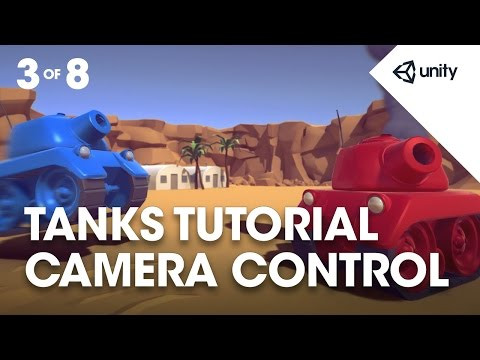# Camera Control

#### -

Phase 3 teaches you how to create a Camera which pans and zooms to keep all tanks onscreen at once.## Camera Control

Intermedio Tanks tutorial

Transcripciones

Phase 3 teaches you how to create a Camera which pans and zooms to keep all tanks onscreen at once.

### Code snippet

``````using UnityEngine;

public class CameraControl : MonoBehaviour
{
public float m_DampTime = 0.2f;                 // Approximate time for the camera to refocus.
public float m_ScreenEdgeBuffer = 4f;           // Space between the top/bottom most target and the screen edge.
public float m_MinSize = 6.5f;                  // The smallest orthographic size the camera can be.
[HideInInspector] public Transform[] m_Targets; // All the targets the camera needs to encompass.

private Camera m_Camera;                        // Used for referencing the camera.
private float m_ZoomSpeed;                      // Reference speed for the smooth damping of the orthographic size.
private Vector3 m_MoveVelocity;                 // Reference velocity for the smooth damping of the position.
private Vector3 m_DesiredPosition;              // The position the camera is moving towards.

private void Awake ()
{
m_Camera = GetComponentInChildren<Camera> ();
}

private void FixedUpdate ()
{
// Move the camera towards a desired position.
Move ();

// Change the size of the camera based.
Zoom ();
}

private void Move ()
{
// Find the average position of the targets.
FindAveragePosition ();

// Smoothly transition to that position.
transform.position = Vector3.SmoothDamp(transform.position, m_DesiredPosition, ref m_MoveVelocity, m_DampTime);
}

private void FindAveragePosition ()
{
Vector3 averagePos = new Vector3 ();
int numTargets = 0;

// Go through all the targets and add their positions together.
for (int i = 0; i < m_Targets.Length; i++)
{
// If the target isn't active, go on to the next one.
if (!m_Targets[i].gameObject.activeSelf)
continue;

// Add to the average and increment the number of targets in the average.
averagePos += m_Targets[i].position;
numTargets++;
}

// If there are targets divide the sum of the positions by the number of them to find the average.
if (numTargets > 0)
averagePos /= numTargets;

// Keep the same y value.
averagePos.y = transform.position.y;

// The desired position is the average position;
m_DesiredPosition = averagePos;
}

private void Zoom ()
{
// Find the required size based on the desired position and smoothly transition to that size.
float requiredSize = FindRequiredSize();
m_Camera.orthographicSize = Mathf.SmoothDamp (m_Camera.orthographicSize, requiredSize, ref m_ZoomSpeed, m_DampTime);
}

private float FindRequiredSize ()
{
// Find the position the camera rig is moving towards in its local space.
Vector3 desiredLocalPos = transform.InverseTransformPoint(m_DesiredPosition);

// Start the camera's size calculation at zero.
float size = 0f;

// Go through all the targets...
for (int i = 0; i < m_Targets.Length; i++)
{
// ... and if they aren't active continue on to the next target.
if (!m_Targets[i].gameObject.activeSelf)
continue;

// Otherwise, find the position of the target in the camera's local space.
Vector3 targetLocalPos = transform.InverseTransformPoint(m_Targets[i].position);

// Find the position of the target from the desired position of the camera's local space.
Vector3 desiredPosToTarget = targetLocalPos - desiredLocalPos;

// Choose the largest out of the current size and the distance of the tank 'up' or 'down' from the camera.
size = Mathf.Max(size, Mathf.Abs(desiredPosToTarget.y));

// Choose the largest out of the current size and the calculated size based on the tank being to the left or right of the camera.
size = Mathf.Max(size, Mathf.Abs(desiredPosToTarget.x) / m_Camera.aspect);
}

// Add the edge buffer to the size.
size += m_ScreenEdgeBuffer;

// Make sure the camera's size isn't below the minimum.
size = Mathf.Max (size, m_MinSize);

return size;
}

public void SetStartPositionAndSize ()
{
// Find the desired position.
FindAveragePosition ();

// Set the camera's position to the desired position without damping.
transform.position = m_DesiredPosition;

// Find and set the required size of the camera.
m_Camera.orthographicSize = FindRequiredSize ();
}
}``````